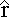#### Field and Potential from Conducting Spheres

We know what the electric field and potential from a point charge look like:

E =
 kQr2and     V =
 kQr

Consider a charged sphere with a symmetrical distribution of charge. Gauss' Law tells us that the electric field outside the sphere is the same as that from a point charge. This implies that outside the sphere the potential also looks like the potential from a point charge.

What about inside the sphere? If the sphere is a conductor we know the field inside the sphere is zero. What about the potential?

Moving from a point on the surface of the sphere to a point inside, the potential changes by an amount:

ΔV = -∫ Eds

Because E = 0, we can only conclude that ΔV is also zero, so V is constant and equal to the value of the potential at the outer surface of the sphere.

#### Potential near an Insulating Sphere

Now consider a solid insulating sphere of radius R with charge uniformly distributed throughout its volume. Once again, outside the sphere both the electric field and the electric potential are identical to the field and potential from a point charge.

What happens inside the sphere? Now the potential is not constant because there is a field inside the sphere. Using Gauss' Law we showed that the field inside a uniformly charged insulator is:

E =
 k Q rR3Use this to calculate the potential inside the sphere. Starting from some point a distance r from the center and moving out to the edge of the sphere, the potential changes by an amount:

ΔV = V(R) - V(r)   =
 R E • ds r
=
 R E dr r
=
 –kQR3
 R r dr r

Integrating gives:

V(R) – V(r) =
 – kQ2R3
(R2 – r2)

V(R) – V(r) =
 – kQ2R
( 1 –
 r2R2
)

V(R) is simply kQ/R, which can be written as 2kQ/2R, so:

V(r) =
 2kQ2R
+
 kQ2R
( 1 –
 r2R2
)

Therefore, for r < R,

V(r) =
 kQ2R
( 3 –
 r2R2
)